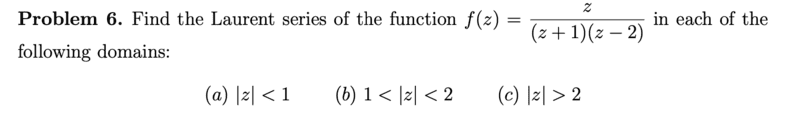# Find the Laurent Series of a function

docnet
Homework Statement:
.
Relevant Equations:
.(a)
i tried to decompose the fracion as a sum of fractions of form ##\frac{1}{1-g}##

$$f=\frac{-z}{(1+z)(2-z)}=\frac{a}{1+z}+\frac{b}{2-z}$$
$$a=\frac{1}{3}, b=-\frac{2}{3}$$

$$f=\frac{1}{6}\frac{1}{1+z}-\frac{1}{3}\frac{1}{1-\frac{z}{2}}$$
$$f=\frac{1}{6}\sum_{n=0}^\infty (-z)^n-\frac{1}{3}\sum_{n=0}^\infty(\frac{z}{2})^n$$

sory i posted too early

Last edited:

docnet
Homework Statement:: .
Relevant Equations:: .

View attachment 293612
(b)

$$f=-\frac{1}{3}\frac{1}{1-\frac{z}{2}}+\frac{1}{3z}\frac{1}{1+\frac{1}{z}}$$
$$f=-\frac{1}{3}\sum_{n=0}^\infty (\frac{z}{2})^n+\frac{1}{3z}\sum_{n=0}^\infty\frac{1}{(-z)^n}$$
then simplify...

(c) $$f=-\frac{2}{3z}\cdot \frac{1}{1-\frac{2}{z}}+\frac{1}{3z}\frac{1}{1+\frac{1}{z}}$$

$$f=-\frac{2}{3z}\sum_{n=0}^\infty (\frac{2}{z})^n+\frac{1}{3z}\sum_{n=0}^\infty\frac{1}{(-z)^n}$$

then simplify...

aside from the need to simplify the expressions, is it a correct idea to re-write the fractions so the sum converges for each part of the problem?

Last edited:
docnet
i don't understand the learning goal of this problem. anyone?

Staff Emeritus
Gold Member
Give people more time to respond!

A Laurent series is an expansion of f into a series of terms ##(z-a)## for some fixed a. They ask you for three series so you probably need to pick three different values of ##a##. Does that help?

•docnet
docnet
Give people more time to respond!

A Laurent series is an expansion of f into a series of terms ##(z-a)## for some fixed a. They ask you for three series so you probably need to pick three different values of ##a##. Does that help?
so even if the terms don't look like ##(z-a)##, it could be the correct expansion of f, right?

is there more than one correct way to expand f into a series over a fixed domain?

docnet
Is the Laurent series another form of the Taylor series?

can inequivalent terms have equivalent sums? like if you have two series expansions of ##f## over a shared domain, but one series expansion has a wider radius of convergence than the other. is there ever a benefit of choosing the series with the smaller radius of convergence? ( is this even possible?)

can one expand any function into a Laurent series?

Staff Emeritus
Homework Helper
aside from the need to simplify the expressions, is it a correct idea to re-write the fractions so the sum converges for each part of the problem?
Yes, you have the right idea.

•docnet
Staff Emeritus
Gold Member
Is the Laurent series another form of the Taylor series?

Yes, except a Laurent series can have terms with negative powers

can inequivalent terms have equivalent sums? like if you have two series expansions of ##f## over a shared domain, but one series expansion has a wider radius of convergence than the other. is there ever a benefit of choosing the series with the smaller radius of convergence? ( is this even possible?)

Yes, you could for example expand ##1/(z-1)## at ##a=0## and get something that converges when ##|z|<1##, or you could expand it around ##a=2/3## and get something that converges when ##1/3 < |z| < 1##. In general the radius of convergence is constrained to points around that are not singularities. On the other hand, if we expand it around ##a=1## we get something that converges for all ##z\neq 1##.

can one expand any function into a Laurent series?

As long as it's well behaved enough, yes.

•docnet
Homework Helper
Gold Member
For the function ##\frac{1}{1-z}##, you can have a Taylor series expanded at ##z_0=0## that converges for ##|z| \lt 1## and a Laurent series centered at ##z_0=0## that converges for ##|z| \gt 1## (since ##\frac{1}{1-z} = -a \frac{1}{1-a}##, where ##a=1/z)##. You can use this concept to combine appropriate series that converge in the regions specified in the problem.

•docnet
Homework Helper
Gold Member
For the function ##\frac{1}{1-z}##, you can have a Taylor series expanded at ##z_0=0## that converges for ##|z| \lt 1## and a Laurent series centered at ##z_0=0## that converges for ##|z| \gt 1## (since ##\frac{1}{1-z} = -a \frac{1}{1-a}##, where ##a=1/z)##. You can use this concept to combine appropriate series that converge in the regions specified in the problem.
Just to complete the explanation:
You already can express the function as the sum of two simple ##\frac{c_1}{c_2-z}## terms.
Each of those can be expanded into a Taylor series that converges in a disk out to its singularity. Call these ##T_1## and ##T_2##.
Also, using the substitution ##a=1/z## in each term, gives a Taylor series in ##a## that converges for ##a## in a disk to its singularity. Substituting the ##1/z## back in for ##a## gives a Laurent series with all negative powers of ##z## that converges for ##z## beyond the singularity. (Note: ##singularity_z = 1/singularity_a##.) Call these ##L_1## and ##L_2##.
For the region of part a of the problem, ##T_1+T_2## is a Taylor series that converges.
For the region of part b of the problem, ##L_1+T_2## is a Laurent series that converges.
For the region of part c of the problem, ##L_1+L_2## is a Laurent series that converges.

Last edited:
•docnet